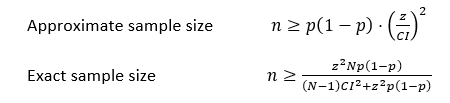# Sample size calculations - exact vs. approximate

Hi all

I am trying to get my head around exact sample size calculations (with population N included) and approximate sample size calculations (based only on outcome rate (p), standard error (se) and confidence interval (CI). Does anyone have a reference or advise on when the approximate sample size calculation would be appropriate over the exact?I will give an example of the issue. We are running a stratified random sample, stratifying by teams within an organisation. The size of each team varies but for the sake of the example let’s say teams have a ‘population’ size of 100, 500 or 1000. We were provided by a consultant a sample size of approximately 300 based on our criteria of p=0.7, CI of 0.05 at a 95% confidence level. Looking at the sample size calculation it is an approximate calculation that doesn’t take into account N (100, 500, 1000). This approach is confusing to me as applying this to a team with 100 people is non-sensical since we technically can’t ‘sample’ 300.

I re-ran this using the exact sample size calculation (including N) and for a population of 100 and 87 is the sample size requried to maintain CI of 0.05 and 95% confidence level, p=0.7 (optimistic but just as an example). So it makes sense to me to calculate the sample sizes based on the exact sample size calculation and include the population size.

Any advice or reference to this issue would be greatly appreciated.